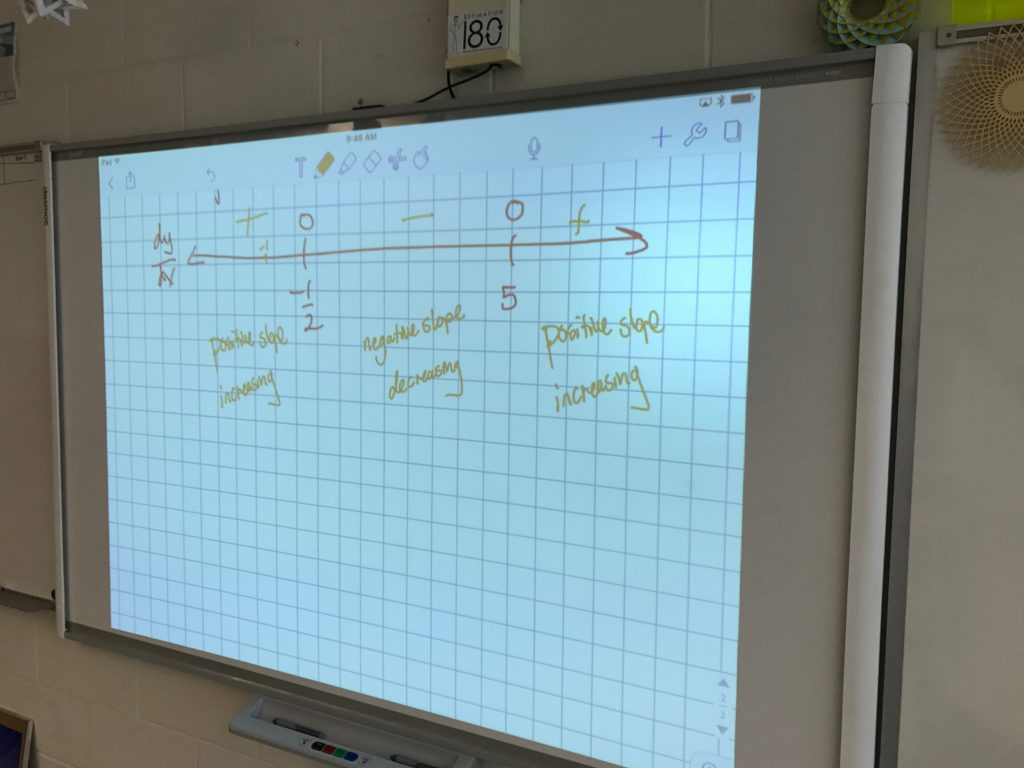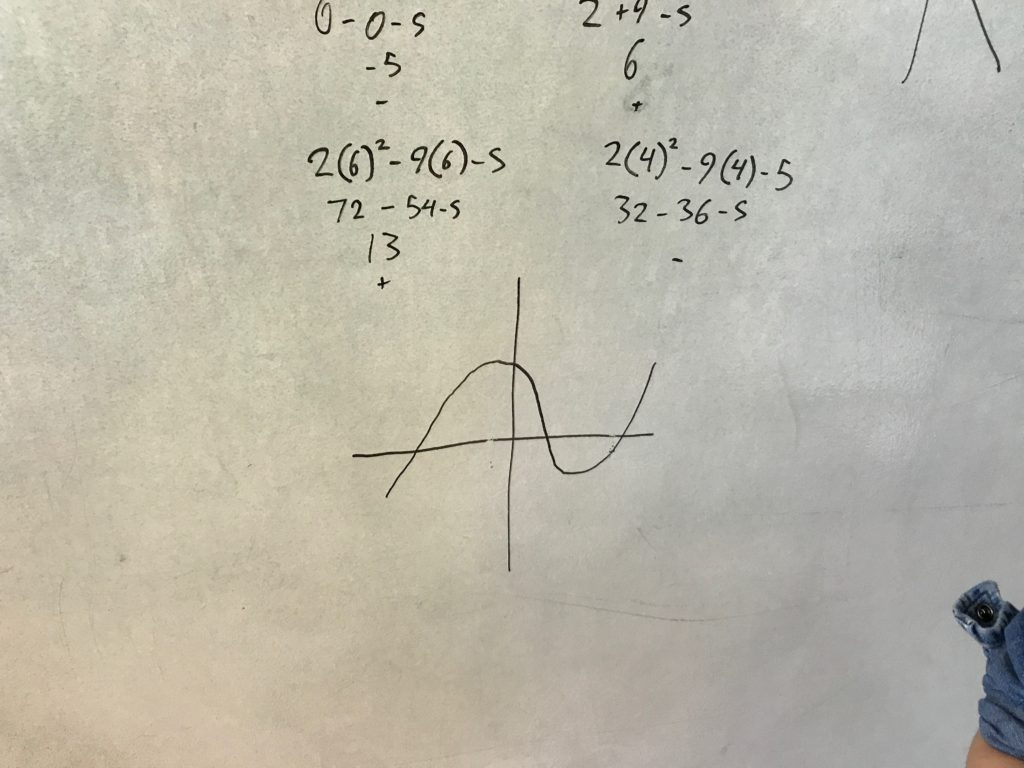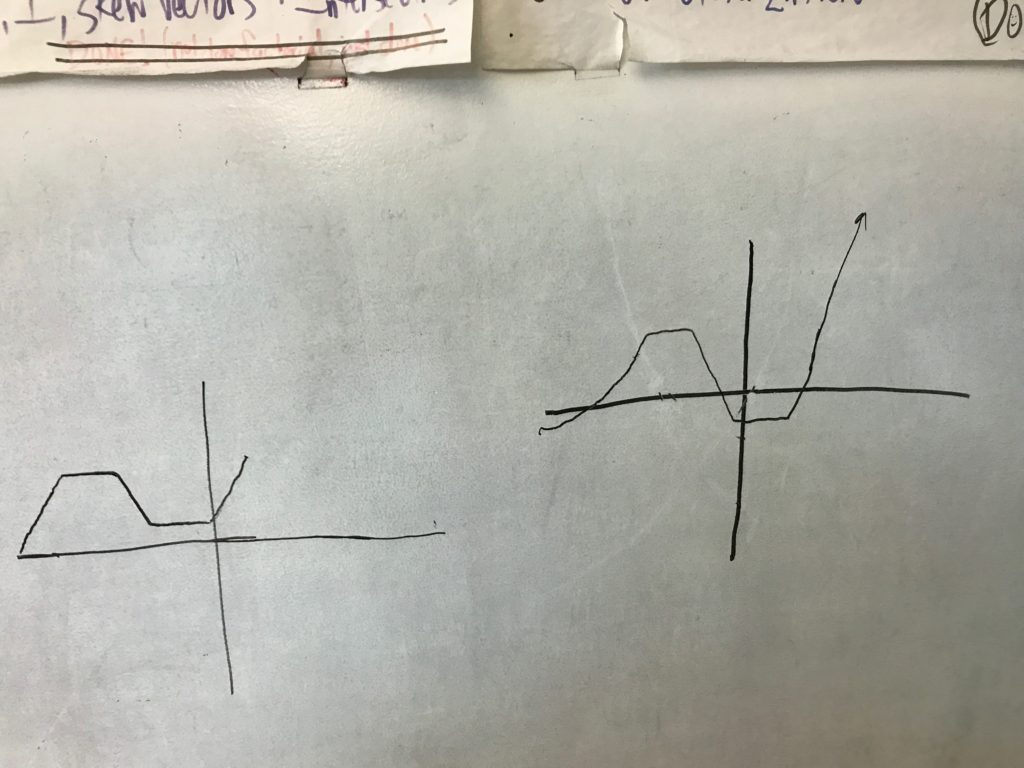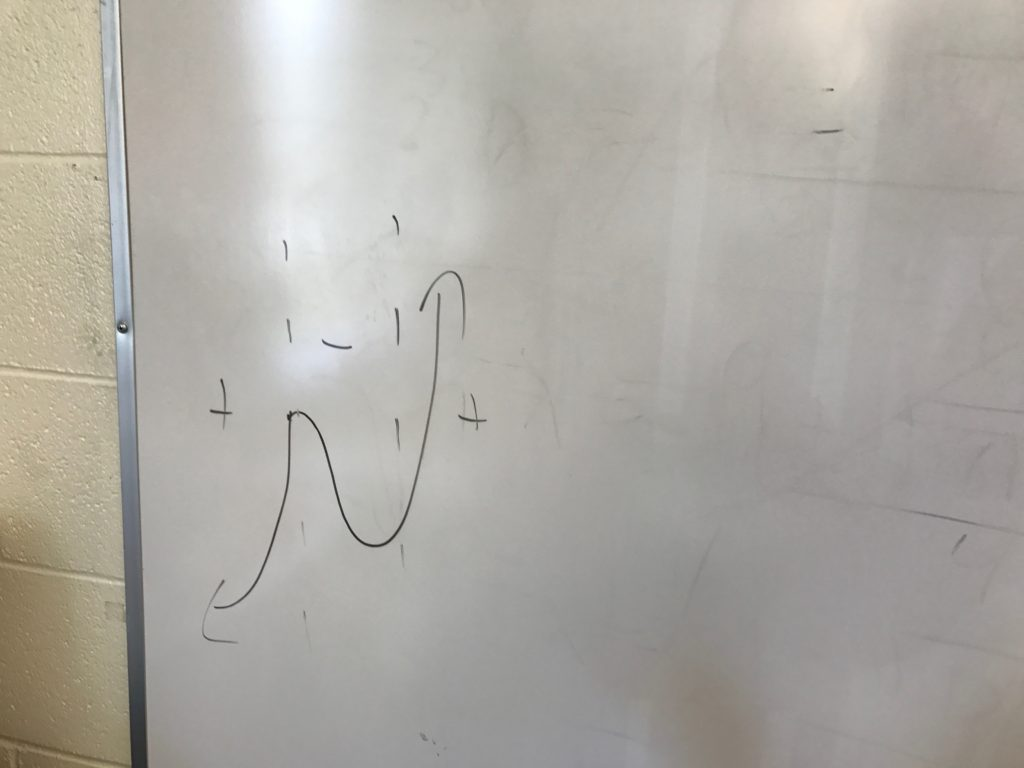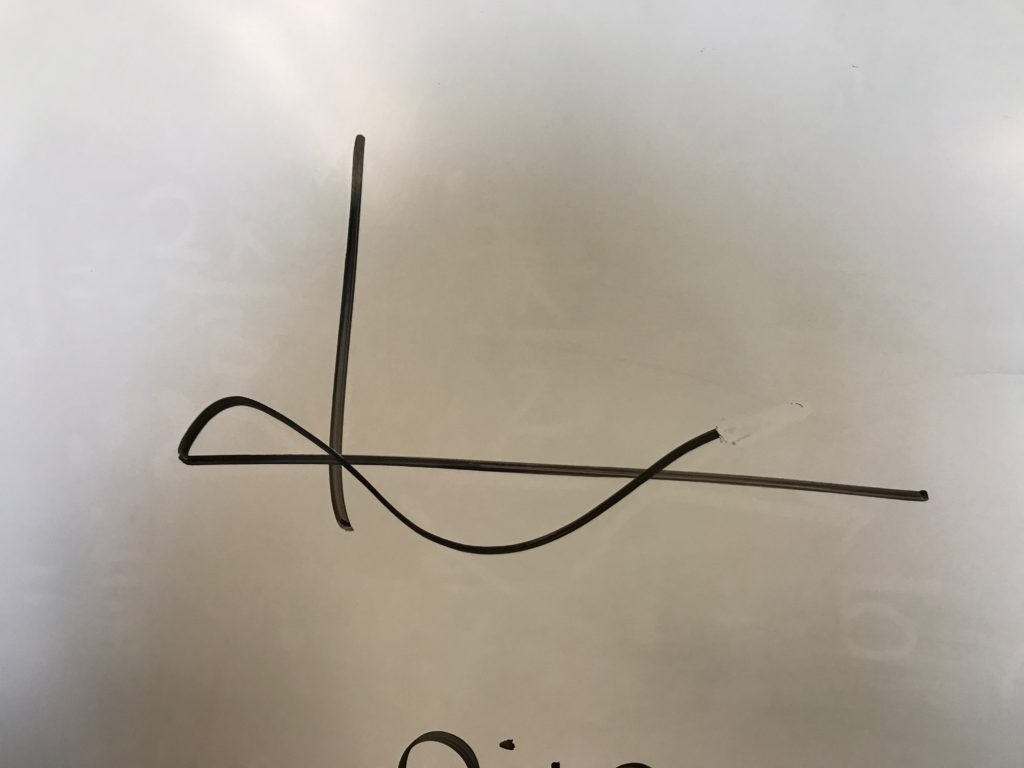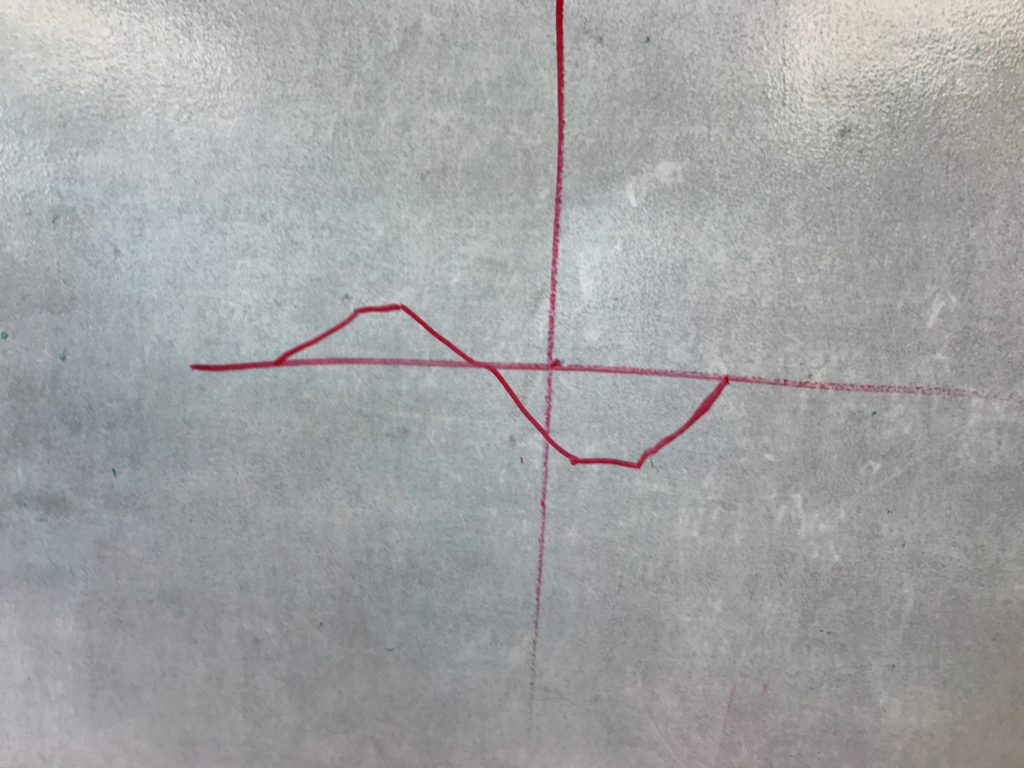As an introduction to curve sketching, the Precalc h class found out where the derivative of a function was zero, positive and negative. Then I had them draw a set of axes and start their marker at the left side of the grid. Told them to start moving the marker to the right, and gave instructions for “increasing”, “decreasing”, or “0 slope”. Asked them, “what do you notice about the spots where the slope was zero?”